• 在DAX表达式中进行除法运算有两个选择，一个是使用DIVIDE函数，另外一个是使用除法操作符，即/（斜杠）。二者在逻辑处理和运算效率上存在一定差别。 使用DIVIDE函数时需要输入两个参数，一个是被除数（分子），另外...
在DAX表达式中进行除法运算有两个选择，一个是使用DIVIDE函数，另外一个是使用除法操作符，即/（斜杠）。二者在逻辑处理和运算效率上存在一定差别。
使用DIVIDE函数时需要输入两个参数，一个是被除数（分子），另外一个是除数（分母）。这两个参数可以是常数，也可以是DAX表达式。由于除法运算中规定除数不能为0，但当除数是某个DAX表达式时，其返回结果可能会出现0或者Null的情况。因此，微软在DIVIDE函数内进行了特殊处理，当被除数为0或Null的情况时，DIVIDE函数会返回Null结果，使得运算可以正常进行下去，而不会中断抛错。DIVIDE函数运算相当于下面这个使用除法操作符的DAX表达式。
Divide =
IF (
OR (
ISBLANK ( [除数] ),
[除数] == 0
),
BLANK (),
[被除数] / [除数]
)


DIVIDE函数内这一特殊处理机制不是简单的使用IF进行判断，而是在运算底层进行了优化，相比这种使用IF函数和除法操作符的DAX表达式效率要高很多。因此，如果除数（分母）是某个DAX表达式时，应该使用DIVIDE函数进行除法运算，以便能获得最好的计算效率。
但如果除数是一个不为0的常量，则应该直接使用除法操作符进行计算。因为在除法操作符这一方法内没有检查除数是否为0的步骤，会对数值直接进行计算，因此在这种情况下，除法操作符的运算效率会比DIVIDE函数的效率高。


展开全文数据分析
• : { $divide: <值> } } 说明 返回字段值除以某个数值的结果。原始值为数组类型时对每个数组元素执行该操作，非数字类型返回 null 。 示例 在集合 sample.employee 插入1条记录： > db.sample.... 语法 { <字段名>: {$divide: <值> } }

说明

返回字段值除以某个数值的结果。原始值为数组类型时对每个数组元素执行该操作，非数字类型返回 null 。

示例

在集合 sample.employee 插入1条记录：

> db.sample.employee.insert( { "a": 300 } )

SequoiaDB shell 运行如下：

作为选择符使用，返回字段“a”除以10的结果：

> db.sample.employee.find( {}, { "a": { "$divide": 10 } } ) { "_id": { "$oid": "582558152b4c38286d00001b"
},
"a": 30
}
Return 1 row(s).

Note: 除数不能为0。

与匹配符配合使用，匹配字段“a”除以10的商为30的记录：

> db.sample.employee.find( { "a": { "$divide": 10, "$et": 30 } } )
{
"_id": {
"$oid": "582558152b4c38286d00001b" }, "a": 300 } Return 1 row(s).  展开全文数据库 • cv::divide()是一个简单的除法函数，有以下两种用法： 用法1： void cv::divide( cv::InputArray src1, // 输入数组1 (分子) cv::InputArray src2, // 输入数组1 (分母) cv::OutputArray dst, // 输出数组 ... cv::divide()是一个简单的除法函数，有以下两种用法： 用法1： void cv::divide( cv::InputArray src1, // 输入数组1 (分子) cv::InputArray src2, // 输入数组1 (分母) cv::OutputArray dst, // 输出数组 (scale*src1/src2) double scale = 1.0, // 乘数因子 int dtype = -1 // 输出数组类型, -1表示与src2相同。 ); 用法2： void cv::divide( double scale, // 所有分子的分母 cv::InputArray src2, // 输入数组 (denominators) cv::OutputArray dst, // 输出数组 (scale/src2) int dtype = -1 // 输出数组类型, -1表示与src2相同。 ); 下面通过两个具体的例子来分别看两种用法的计算效果： 用法1：  cv::Mat img0 = cv::imread("img_0.jpg", -1); cv::Mat img1 = cv::imread("img_1.jpg", -1); if (img0.empty()) { std::cout << "load img0 failed!\n" << std::endl; return -1; } if (img1.empty()) { std::cout << "load img1 failed!\n" << std::endl; return -1; } cv::Mat img2; cv::divide(img0, img1, img2, 50, -1); cv::imshow("img0", img0); cv::imshow("img1", img1); cv::imshow("img2", img2); cv::waitKey(0); return 0; 结果如下：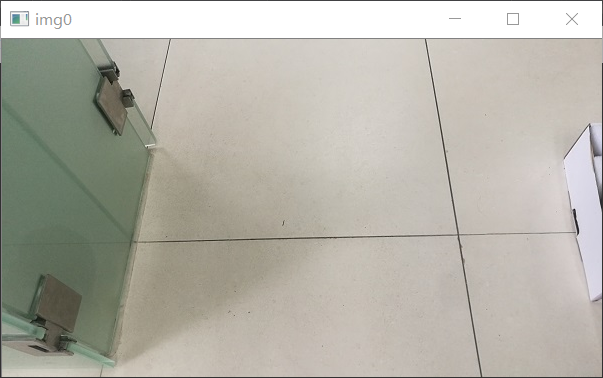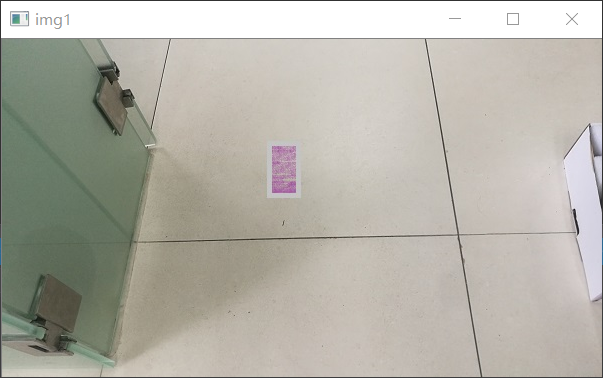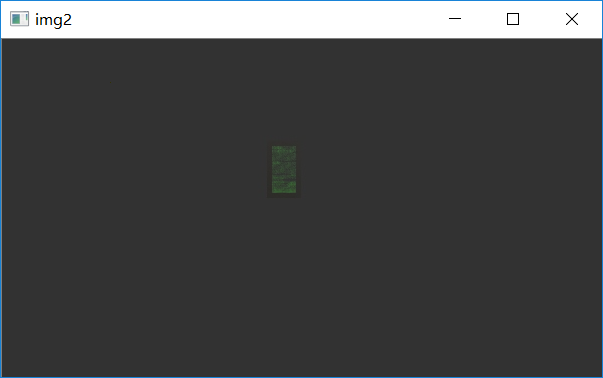用法2：  cv::Mat img0 = cv::imread("img_1.jpg", -1); if (img0.empty()) { std::cout << "load img0 failed!\n" << std::endl; return -1; } cv::Mat img1; cv::divide(5000, img0, img1, -1); cv::imshow("img0", img0); cv::imshow("img1", img1); cv::waitKey(0); return 0; 结果如下：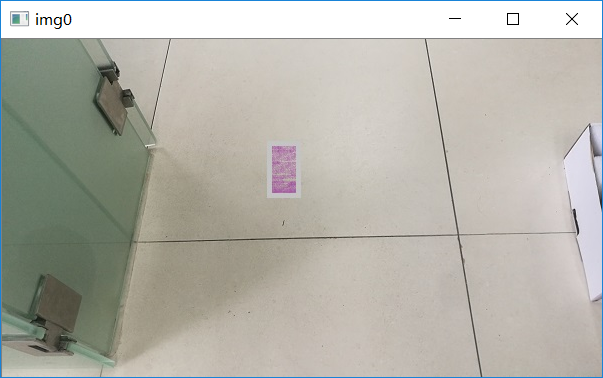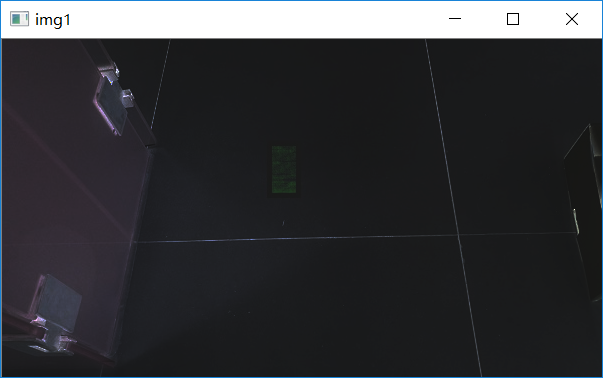展开全文• cv::divide() 两个数组逐元素相除 cv::eigen() 计算方形矩阵的特征值和特征向量 cv::exp() 逐元素求指数 cv::filp() 翻转矩阵 cv::gemm() 两个数组逐元素相乘 cv::filp() 翻转矩阵 ... 1、目录 cv::determinant() 计算方形矩阵的行列式 cv::divide() 两个数组逐元素相除 cv::eigen() 计算方形矩阵的特征值和特征向量 cv::exp() 逐元素求指数 cv::filp() 翻转矩阵 cv::gemm() 两个数组逐元素相乘 cv::filp() 翻转矩阵 cv::gemm() 两个数组逐元素相乘 2、例子代码 //包含OpenCV的头文件 #include <opencv2/opencv.hpp> #include <opencv2/core/mat.hpp> #include <iostream> #include <time.h> using namespace std; //使用OpenCV的命名空间 using namespace cv; //测试cvtColor //转换空间为Y=0.299R + 0.587G + 0.114B //测试determinant() //计算矩阵的行列式 //需要主要的地方是：必须是方形矩阵，必须浮点型数据并且是单通道类型 /* Matrix m is: [1, 2; 3, 4] determinant is: -2 */ void test_determinant() { const int iRows = 2; const int iCols = 2; Mat m(iRows, iCols, CV_32FC1); m.at<float>(0, 0) = 1; m.at<float>(0, 1) = 2; m.at<float>(1, 0) = 3; m.at<float>(1, 1) = 4; double value = determinant(m); cout << "Matrix m is:\n" << m << endl; cout << "determinant is:\t" << value << endl; return; } //测试divide() //两个矩阵逐像素相除 //CV_EXPORTS_W void divide(InputArray src1, InputArray src2, OutputArray dst,double scale = 1, int dtype = -1); //公式 scale*src1/src2; //src1 输入矩阵 //src2 第二个输入矩阵；第二个矩阵和第一个矩阵大小是相同的，并且类型也是相同的 //dst 输出矩阵 //scale 缩放因子 //dtype 输出影像的深度，注意的地方：如果传入-1的话，那么输出矩阵的深度和src2是相同的 //还有另外一个函数 //CV_EXPORTS_W void divide(double scale, InputArray src2,OutputArray dst, int dtype = -1); //公式不同的地方在于 //scale/src2 /* Matrix src1 is: [1, 2, 3; 4, 5, 6; 7, 8, 9] Matrix src2 is: [9, 8, 7; 6, 5, 4; 3, 2, 1] Result matrix is: [0.11111111, 0.25, 0.42857143; 0.66666669, 1, 1.5; 2.3333333, 4, 9] Result1 matrix is: [1, 0.5, 0.33333334; 0.25, 0.2, 0.16666667; 0.14285715, 0.125, 0.11111111] */ void test_divide() { const int iRows = 3; const int iCols = 3; Mat src1(iRows, iCols, CV_32FC1); Mat src2(iRows, iCols, CV_32FC1); //测试第一个divide int Index = 1; for (int i=0;i<iRows;++i) { for (int j=0;j<iCols;++j) { src1.at<float>(i, j) = Index; src2.at<float>(i, j) = 10 - Index; ++Index; } } cout << "Matrix src1 is:\n" << src1 << endl; cout << "Matrix src2 is:\n" << src2 << endl; Mat result; divide(src1, src2, result, 1, CV_32F); cout << "Result matrix is:\n" << result << endl; //测试第二个divide Mat result1; divide(1.0, src1,result1, 1, CV_32F); cout << "Result1 matrix is:\n" << result1 << endl; return; } //测试eigen() //计算特征值 //需要注意的地方：矩阵必须为float类型,并且是对称矩阵，非对称矩阵的话，计算出来的是错误的 /* Matrix m is: [2, 1, 0; 1, 3, 1; 0, 1, 2] eigen values is: [3.9999998; 1.9999999; 1.0000001] eigen vectors is: [0.40824822, 0.81649661, 0.40824834; -0.70710671, -4.2503757e-08, 0.70710689; 0.57735038, -0.57735038, 0.57735026] */ void test_eigen() { const int iRows = 3; const int iCols = 3; Mat m(iRows, iCols, CV_32FC1); m.at<float>(0, 0) = 2; m.at<float>(0, 1) = 1; m.at<float>(0, 2) = 0; m.at<float>(1, 0) = 1; m.at<float>(1, 1) = 3; m.at<float>(1, 2) = 1; m.at<float>(2, 0) = 0; m.at<float>(2, 1) = 1; m.at<float>(2, 2) = 2; //计算特征值和特性向量 Mat eigenValues, eigenVectors; eigen(m, eigenValues, eigenVectors); cout << "Matrix m is:\n" << m << endl; cout << "eigen values is:\n" << eigenValues << endl; cout << "eigen vectors is:\n" << eigenVectors << endl; return; } //测试exp() //计算指数值 /* source matrix is: [0, 0, 0; 1, 1, 1; 2, 2, 2] Result matrix is: [1, 1, 1; 2.718282, 2.718282, 2.718282; 7.3890557, 7.3890557, 7.3890557] */ void test_exp() { const int iRows = 3; const int iCols = 3; Mat m(iRows, iCols, CV_32FC1); for (int i=0;i<iRows;++i) { m.at<float>(i, 0) = m.at<float>(i, 1) = m.at<float>(i, 2) = i; } Mat Result; exp(m, Result); cout << "source matrix is:\n" <<m<< endl << endl; cout << "Result matrix is:\n" << Result << endl << endl; return; } //测试flip() //CV_EXPORTS_W void flip(InputArray src, OutputArray dst, int flipCode); //src 输入矩阵 //dst 输出矩阵 //flipCode 如果大于0的话，沿Y轴进行翻转；如果等于0的话，沿X轴进行翻转；如果小于0的话，那么同时沿X，Y进行翻转 /* source matrix is: [ 91, 2, 79; 179, 52, 205; 236, 8, 181] matrix flip Y axis is: [ 79, 2, 91; 205, 52, 179; 181, 8, 236] matrix flip X axis is: [236, 8, 181; 179, 52, 205; 91, 2, 79] matrix flip X Y axis is: [181, 8, 236; 205, 52, 179; 79, 2, 91] */ void test_flip() { const int iRows = 3; const int iCols = 3; Mat src(iRows, iCols, CV_8UC1); randu(src, 0, 255); cout << "source matrix is:\n" << src << endl << endl; Mat dst; flip(src, dst, 1); cout << "matrix flip Y axis is:\n" << dst << endl << endl; flip(src, dst, 0); cout << "matrix flip X axis is:\n" << dst << endl << endl; flip(src, dst, -1); cout << "matrix flip X Y axis is:\n" << dst << endl << endl; return; } //测试inRange() //CV_EXPORTS_W void inRange(InputArray src, InputArray lowerb,InputArray upperb, OutputArray dst); //如果在范围之内的话，设置值为255，不然的话结果为0；并且输出矩阵的深度为CV_8U //这个范围为[lower,upper] /* source matrix is: [ 1, 2, 3; 4, 5, 6; 7, 8, 9] inRange matrix is: [ 0, 0, 255; 255, 255, 255; 0, 0, 0] */ void test_inRange() { const int iRows = 3; const int iCols = 3; Mat src(iRows, iCols, CV_8UC1); int Index = 1; for (int i = 0; i < iRows; ++i) { for (int j = 0; j < iCols; ++j) { src.at<unsigned char>(i, j) = Index; ++Index; } } cout << "source matrix is:\n" << src << endl << endl; Mat dst; inRange(src, 3, 6, dst); cout << "inRange matrix is:\n" << dst << endl << endl; return; } //测试invert() //CV_EXPORTS_W double invert(InputArray src, OutputArray dst, int flags = DECOMP_LU); //src 输入矩阵，注意这个矩阵必须是浮点型的 //dst 输出矩阵 //三个标志 DECOMP_LU DECOMP_SVD DECOMP_CHOLESKY //如果矩阵是n*m 的话，那么返回的结果为m*n //在使用LU分解的话，返回值为src的行列式，如果行列式失败的话，结果为0 //SVD 分解返回出来的结果为最小特征值和最大特征值的比例 //注意的地方：LU和CHOLSKY 分解必须是方形矩阵，非奇异矩阵，并且是正定矩阵 /* source matrix is: [1, 2, 3; 1, 0, -1; 0, 1, 1] invert matrix is: [0.5, 0.5, -1; -0.5, 0.5, 2; 0.5, -0.5, -1] */ void test_invert() { const int iRows = 3; const int iCols = 3; Mat src(iRows, iCols, CV_32FC1); src.at<float>(0, 0) = 1; src.at<float>(0, 1) = 2; src.at<float>(0, 2) = 3; src.at<float>(1, 0) = 1; src.at<float>(1, 1) = 0; src.at<float>(1, 2) = -1; src.at<float>(2, 0) = 0; src.at<float>(2, 1) = 1; src.at<float>(2, 2) = 1; cout << "source matrix is:\n" << src << endl << endl; Mat dst; invert(src, dst, DECOMP_LU); cout << "invert matrix is:\n" << dst << endl << endl; return; } //测试log //注意的地方：log值应该是大于0的值 /* source matrix is: [-3, -2, -1; 0, 1, 2; 3, 4, 5] log matrix is: [-nan(ind), -nan(ind), -nan(ind); -inf, 0, 0.69314718; 1.0986123, 1.3862944, 1.6094379] */ void test_log() { const int iRows = 3; const int iCols = 3; Mat src(iRows, iCols, CV_32FC1); int Index = -3; for (int i = 0; i < iRows; ++i) { for (int j = 0; j < iCols; ++j) { src.at<float>(i, j) = Index; ++Index; } } cout << "source matrix is:\n" << src << endl << endl; Mat dst; log(src, dst); cout << "log matrix is:\n" << dst << endl << endl; return; } int main() { //修改默认的随机数 //RNG rng(time(NULL)); //theRNG() = rng; test_log(); return 0; }   展开全文• py 清除函数缓存代码Functions are an integral part of programming. They help add modularity and reusability to our code. 函数是编程的组成部分。...It’s quite common to divide our program into chunks...python java javascript js 人工智能 • #define _CRT_SECURE_NO_WARNINGS #include <stdio.h> #include <stdlib.h> #include <string.h> int add(int a, int b) { return a + b; } int sub(int a, int b) ...int divideC 框架 多态 • 要在浏览器中使用，请使用 。 用法 var divide = require ( 'compute-divide' ) ; 除法（arr，x [，opts]） 计算按元素划分。 x可以是长度相等的array ，也可以是numeric 。 var arr = [ 2 , 1 , 4 , 2 ] , ...JavaScript • 该骨架在构造函数中采用4个lambda，分别代表divide ， combine ， base和threshold函数。然后，框架解决问题并自动处理负载平衡，工作窃取和任务依赖性。 被用作启发。 怎么跑 cd src make ./mainC++ • 1.array_divide() array_divide 函数返回两个数组，一个包含原始数组的健，另一个包含原始数组的值 [$keys, \$values] = array_divide(['name' =&...array_dot 函数将多维数组平铺到一维数组中，该数组使用「点...
• Laravel有很多全局函数来帮助我们更高效地编程，这些函数都是我们经常可能会使用到的： Available Methods Arrays array_add array_collapse array_divide array_dot array_except array_first array_...url laravel global previous 返回
• 实现了一个正整数除法，使用加减和移位完成，然后将所有整数除法分类处理进入正整数除法函数内。 主要是要注意边界INT_MIN的特殊性。 代码： class Solution { public: int divide_inner(int dividend, int divisor...LeetCode C++ 算法
• 使用def语句创建函数：def remainder(a, b): q = a // b # // 是截断除法运算符　r = a - q*b return r1.11.1 调用函数：result = remainder(5,6)1.11.2 使用元组返回多个值def divide(a, b): q = a // b # // 是...
• 定义方式：int (*p)(int x, int y);#include int sum(int x, int y){ return x + y; } int reduce(int x, int y){ return x - y; } int multiply(int x, int y){ return x * y;...float divide(inc语言 指针
• DIVIDE函数 作用： 安全除法，一个值得使用的除法函数。 在分母为0时，返回备用结果或BLANK() 语法： DIVID(<numerator>, <denominator>[,<alternateresult>]> 参数： numerator 分子 ...
• 思路： 随机选取列表中的一个值v，然后将列表分为小于v的，等于v的，大于v的三组。对于k<=left.size()时， 在left中执行selection；...需要注意rand()函数使用。 #include <iostream> #in...
• 给定两个整数的被除数和除数，在不使用乘法、除法和余运算符的情况下对两个整数进行除法；返回商；向零截断。 被除数和除数都是32位带符号整数；除数永远不会是0 假设我们处理的环境只能存储32位带符号整数范围内...
• //变量的提升 （不推荐） x=6; console.log(x);//使用 var x;//在使用之后声明 //函数的提升 （推荐） console.log(divide(8,4));//使用 function divide(a,b){//在使用之后声明 return a/b; }
• 使用Python对神经网络进行编程实现的时候，往往由于设置的学习率α偏大，或者由于在对W进行np.random.randn()的时候，会遭遇系统报RuntimeWarning: divide by zero encountered in log错误。 下面的定义的激活函数...深度学习 神经网络
• 通过函数传递值，这是为ES使用（|>）的一种替代方法。 。 也支持返回承诺的功能。 在这种情况下，连锁的结果也将是一个希望。 这类似于在链式功能中为等待提供的拟议支持。 安装 npm install ppipe ppipe解决的...
• #include#includeint _add(int a,int b){return a+b;}int _minus(int a,int b){return a-b;}int _muti(int a,int b){return a...}double divide(int a,int b){if(!b){printf("Error!\n");exit(1);}return (double)a/...
• 首先使用function函数需要#include 个人现在看类似于自定义一个类型名，类似于auto，int，string这样的。 比如： // 普通函数 int add(int i, int j) { return i + j; } // lambda表达式 auto mod = [](int i, int...c++
• BigDecimal的初始化：BigDecimal num = new BigDecimal(); 函数： 1.加法函数: add() 2.减法函数：substract() ...4.除法函数divide(） 使用方法： //加法 BigDecimal result1 = num1.add(num2); ...
• 大家都知道函数返回值可以返回数据给调用者，也有必要掌握使用指针变量作为函数参数充当函数返回值的方法。请你帮助叮当定义形如下面的函数： void fun(int a, int b, int *sum, int *diff, int *product, float *...
• 前言 ...遵循这种思想我们可以将数学上的中缀表达式”(56-9)*3+1/6”使用函数的方式来表示： ADD(MULTIPLY(MINUS(56,9),3),DIVIDE(1,6)) 即每一种运算符都可以抽象为一个函数（比较类似于前缀表达结构 语言 c# 解释器
• 分治法，从字面上理解即为“分而治之”。分而治之，即将复杂的问题分解为简单的子问题，再把子问题分解为更小的问题，直到问题可以直接得到解答。原问题的解即为子问题解的合并。...使用递归往往可以使函数的定义和...
• TensorFlow 提供了几种操作，您可以使用它们将基本算术运算符添加到图形中。 tf.add tf.subtract tf.multiply tf.scalar_mul tf.div tf.divide tf.truediv tf.floordiv tf.realdiv tf.truncatediv tf....tensorflow
• std::bind将可调用对象与其参数一起进行绑定，绑定后的结果可以使用std::function保存。 作用： 将可调用对象和其参数绑定成一个防函数； 只绑定部分参数，减少可调用对象传入的参数（可用lambda匿名函数包裹原函数...
• 在Go中，函数类型是一等类型，这意味着可以把函数当作一个值来传递和使用。函数值既可以作为其他函数的参数，也可以作为其结果。另外，我们还可以利用函数类型的这一特性生成闭包。一个函数的声明通常包括关键字func...
• 快速排序是东尼霍尔发现的一种排序算法。在平均情况下，排序n个项目要O(nlogn)O(nlogn)O(nlogn)次...快速排序使用分治法（Divide and Conquer）策略来把一个串行（list）分为两个子串行（sub-lists）。 快速排序又是一快速排序 排序算法 java 算法...